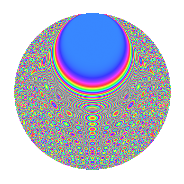# Properties

 Label 2016.2.dmLevel 2016 Weight 2 Character orbit dm Rep. character $$\chi_{2016}(253,\cdot)$$ Character field $$\Q(\zeta_{8})$$ Dimension 480 Sturm bound 768

# Related objects

## Defining parameters

 Level: $$N$$ = $$2016 = 2^{5} \cdot 3^{2} \cdot 7$$ Weight: $$k$$ = $$2$$ Character orbit: $$[\chi]$$ = 2016.dm (of order $$8$$ and degree $$4$$) Character conductor: $$\operatorname{cond}(\chi)$$ = $$32$$ Character field: $$\Q(\zeta_{8})$$ Sturm bound: $$768$$

## Dimensions

The following table gives the dimensions of various subspaces of $$M_{2}(2016, [\chi])$$.

Total New Old
Modular forms 1568 480 1088
Cusp forms 1504 480 1024
Eisenstein series 64 0 64

## Trace form

 $$480q + O(q^{10})$$ $$480q + 16q^{10} - 4q^{16} + 36q^{22} - 8q^{23} + 40q^{26} + 40q^{32} + 40q^{34} + 56q^{38} + 40q^{40} - 8q^{43} + 4q^{44} + 64q^{46} + 104q^{50} + 64q^{52} - 16q^{53} - 64q^{55} + 28q^{56} + 72q^{58} + 64q^{61} + 72q^{62} + 8q^{67} - 8q^{68} - 48q^{70} + 28q^{74} - 32q^{76} - 16q^{77} - 144q^{80} - 80q^{82} - 168q^{86} - 80q^{88} - 156q^{92} - 152q^{94} + 128q^{95} + O(q^{100})$$

## Decomposition of $$S_{2}^{\mathrm{new}}(2016, [\chi])$$ into newform subspaces

The newforms in this space have not yet been added to the LMFDB.

## Decomposition of $$S_{2}^{\mathrm{old}}(2016, [\chi])$$ into lower level spaces

$$S_{2}^{\mathrm{old}}(2016, [\chi]) \cong$$ $$S_{2}^{\mathrm{new}}(32, [\chi])$$$$^{\oplus 6}$$$$\oplus$$$$S_{2}^{\mathrm{new}}(96, [\chi])$$$$^{\oplus 4}$$$$\oplus$$$$S_{2}^{\mathrm{new}}(224, [\chi])$$$$^{\oplus 3}$$$$\oplus$$$$S_{2}^{\mathrm{new}}(288, [\chi])$$$$^{\oplus 2}$$$$\oplus$$$$S_{2}^{\mathrm{new}}(672, [\chi])$$$$^{\oplus 2}$$

## Hecke Characteristic Polynomials

There are no characteristic polynomials of Hecke operators in the database